WORKSHEET: FRACTIONS 1 Worksheets Descargar PDF 1) What fraction of each grid is IN GREEN? a)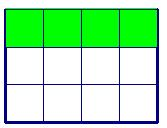b)c)Solution 2) What fraction of each grid is IN YELLOW? a)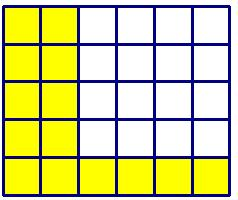b)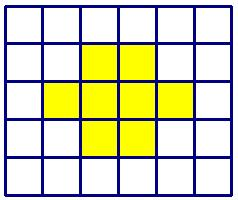c)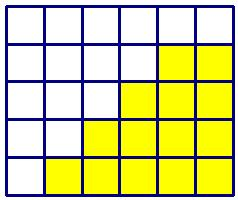Solution 3) What fraction of each shape is shaded? a)b)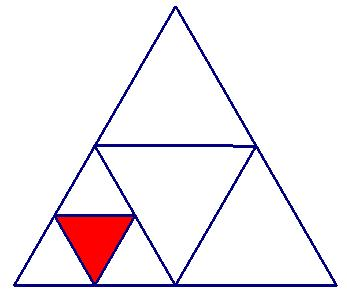c)Solution 4) Divide each cross into the given fraction: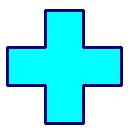HALVESQUARTERSFIFTHSEIGHTHS Solution 5)Four kids have a pizza to share equally. What fraction of pizza did each kid get?Solution 6) There are 12 shapes in a box and 9 of them are cubes. What fraction of shapes are cubes?Solution 7) Bob has 18 marbles and he has to give 2/3 of them to Karen. How many marbles Karen got from Bob?Solution 8) Lea and her dad have to paint 12 metres wall. They finished 3/4 of the wall. They painted _________ m of wall. What fraction of wall is left to paint?Solution 9) What fraction of 1 hour is 15 minutes? And 12 minutes? And 8 minutes?Solution 10) Two-thirds of a fish weighs 10 pounds. How heavy is the whole fish?Solution